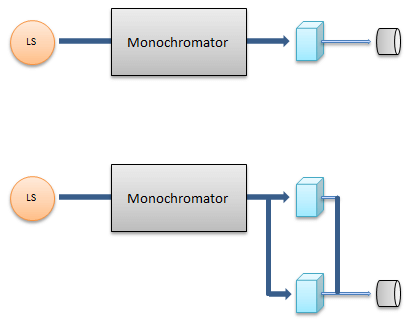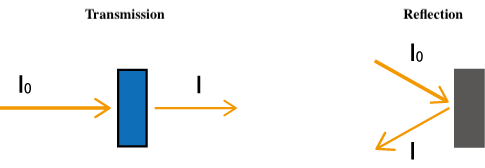# Principles of UV/vis spectroscopy (2) Basics of absorption spectroscopy

October 6, 2020

## Absorption spectrophotometer

A UV-vis spectrophotometer comprises a light source, a monochromator, a sample cuvette (cell), and a photodetector. There are two types of optical arrangement: single beam and double beam (Fig. 4). In either case, white light from a light source (LS) is introduced into a monochromator and dispersed by a diffraction grating. Only light with a specific wavelength is extracted from the resulting spectrum through the exit slit. In the case of a single beam, the obtained monochromatic light with intensity I0 irradiates a sample and the transmitted light with intensity I is detected. Here, I/I0 is the transmittance. In the double-beam configuration, the monochromatic light is divided into two by a beam splitter, and the individual beams pass through a sample and a reference, following which both are detected. By bifurcating the optical path, both I0 and I can be measured simultaneously.Fig. 4 UV-vis spectrophotometer configuration (upper: single beam, lower: double beam)

## Beer's law

Beer's law forms the basis of quantification using absorption spectroscopy. Here, the intensity of incident light in a sample cuvette is I0 and the intensity of transmitted light is I, and

I = I0 e -εC L

where C is the molar concentration of the solution, L is the thickness, and ε is the molar extinction coefficient
Taking the logarithm on both sides and transforming the formula,

-log (I / I0 ) = εCL

If the left side -log(I / I0) is defined as the absorbance A, then

A = εCL

That is, the absorbance A is proportional to the concentration C of the sample (Fig. 5). This equation is a calibration curve, and it can be used to accurately determine the concentration of unknown substances.Fig. 5 Calibration curve for quantitative analysis

## Photometric mode

The photometric modes (the vertical axis of the spectrum) used in UV-vis spectroscopy are:

Absorbance: Abs = log (I0 / I)
Transmittance: %T = I / I0 × 100
Reflectance: %R = I / I0 × 100

The experimental setup for transmission and reflection measurements is shown in Fig. 6.

When measuring a sample, Abs is used for quantitative analysis of factors such as concentration and turbidity, and %T and %R are used to determine factors such as the transmission/reflection characteristics, film thickness, and color.Fig. 6 Transmission measurement (%T) and reflection measurement (%R)